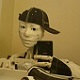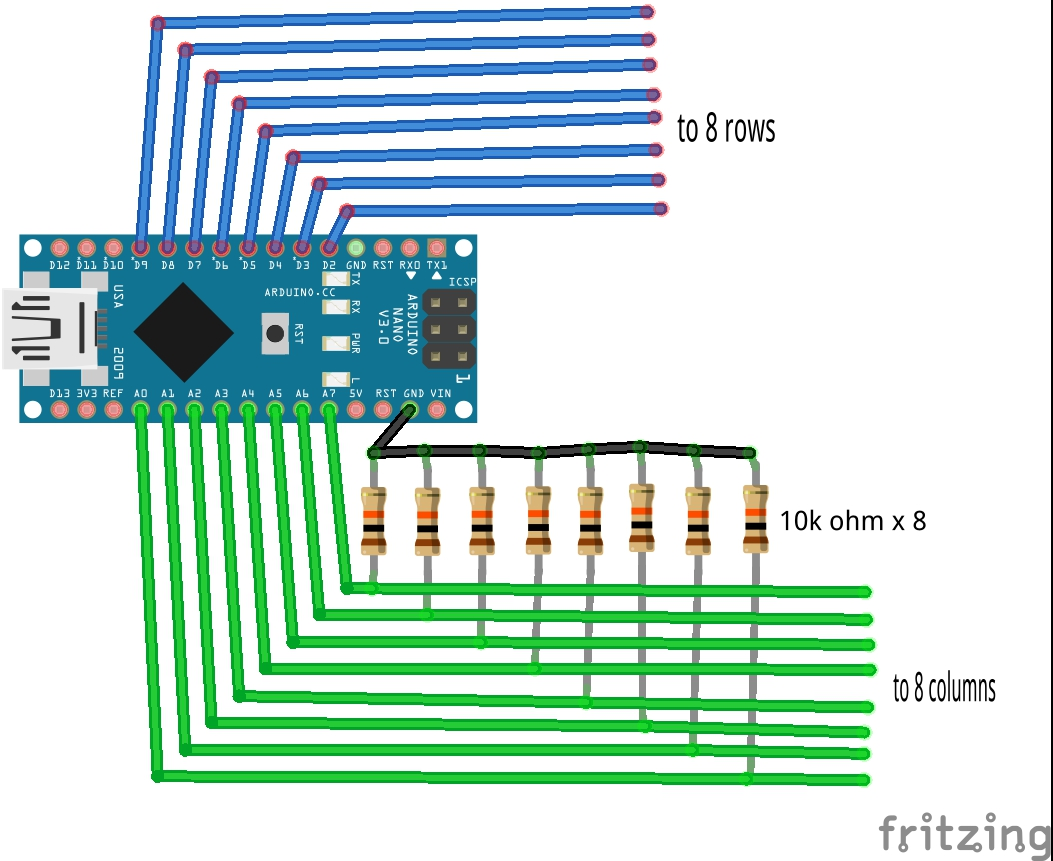# Touch sensitive robotic skin...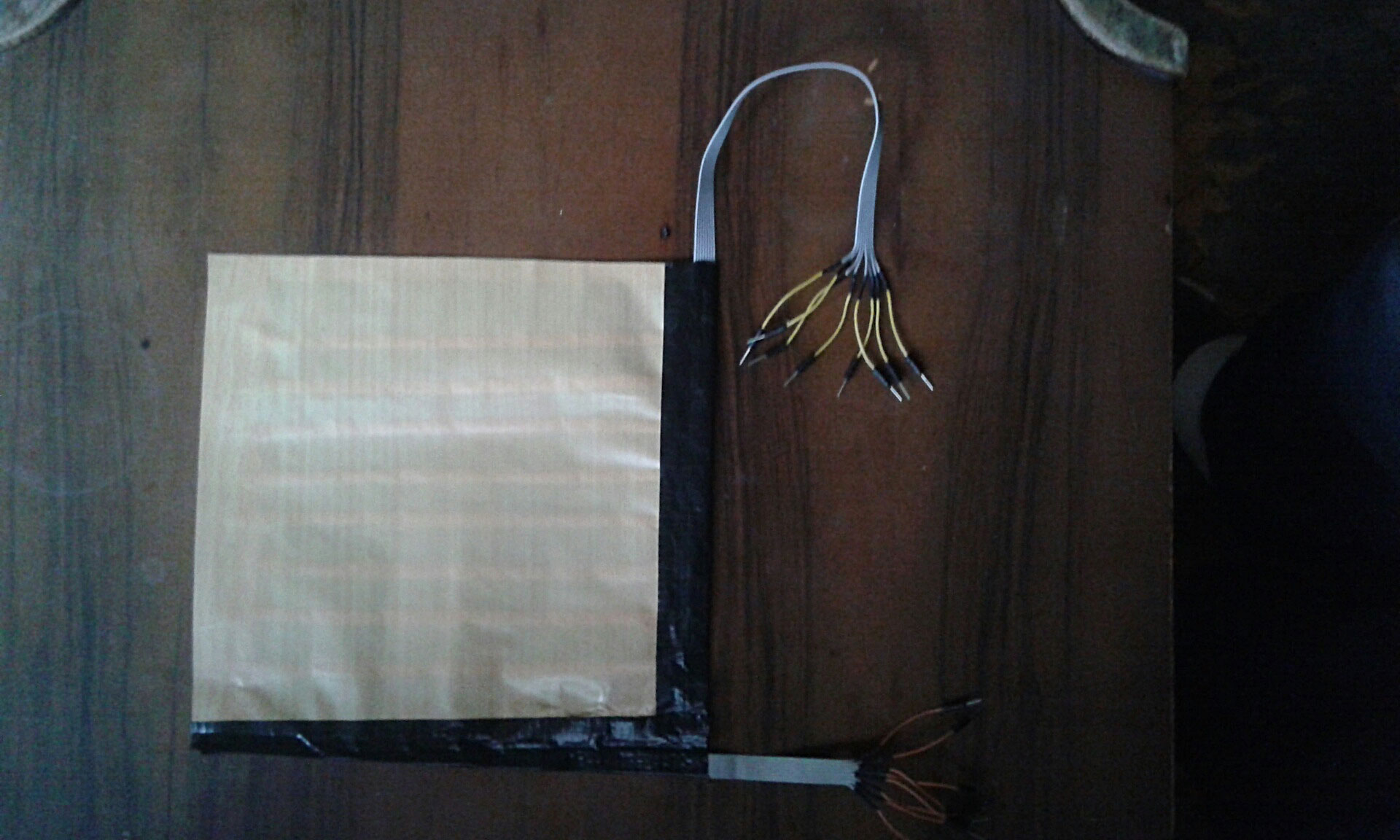Inspired by the work of Markus and having a lot of background on DIY resistive sensors, I decided to make a different approach to creating touch sensitive robotic skin... The skin pad you see on above pic shows a pad 20cmx20cm 8bit matrix with 8x8=64 junction points controlled with 8 analog and 8 digital pins on Arduino... I made this matrix inspired with the work of Markus and another study here  and here ... Check the below pics for the materials and construction...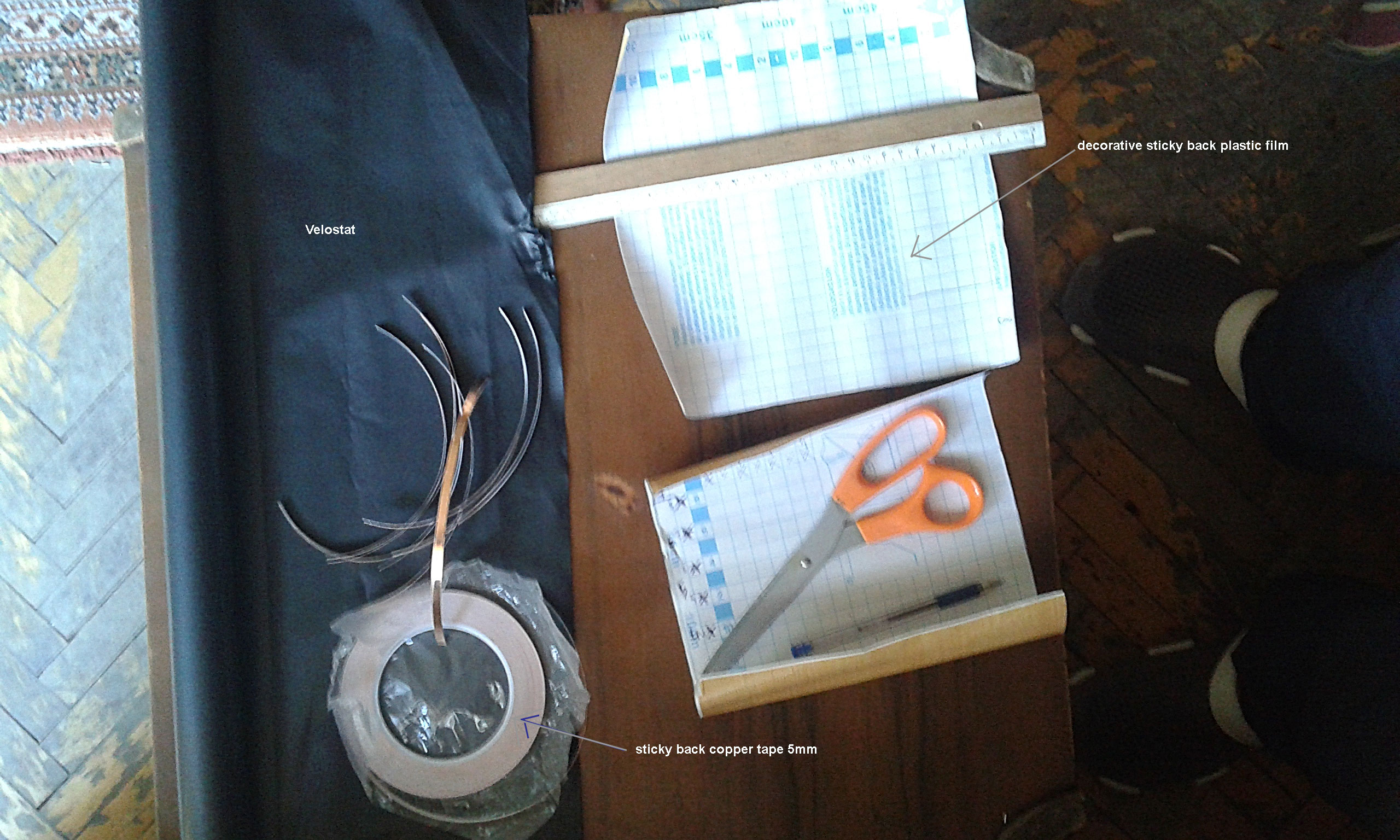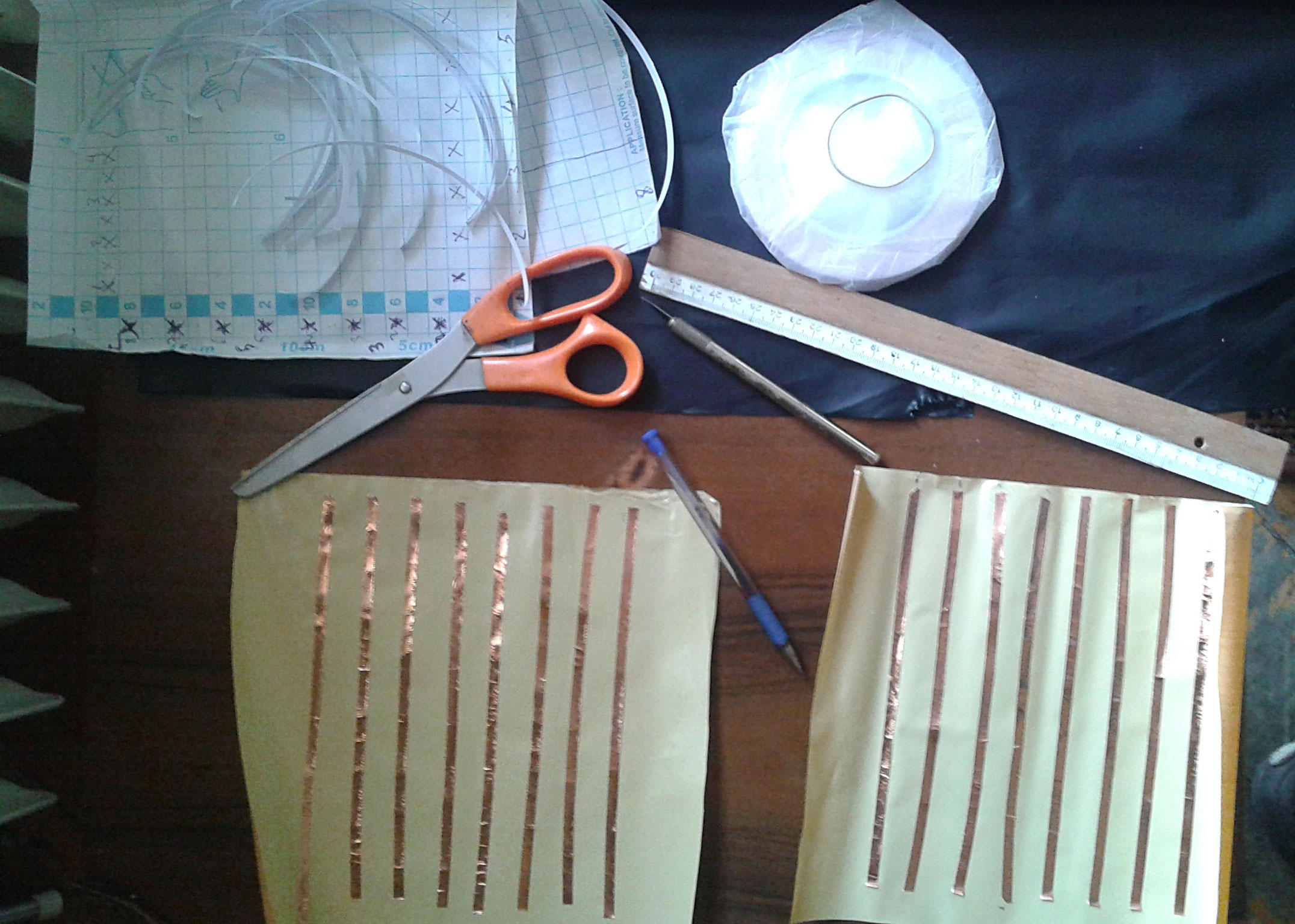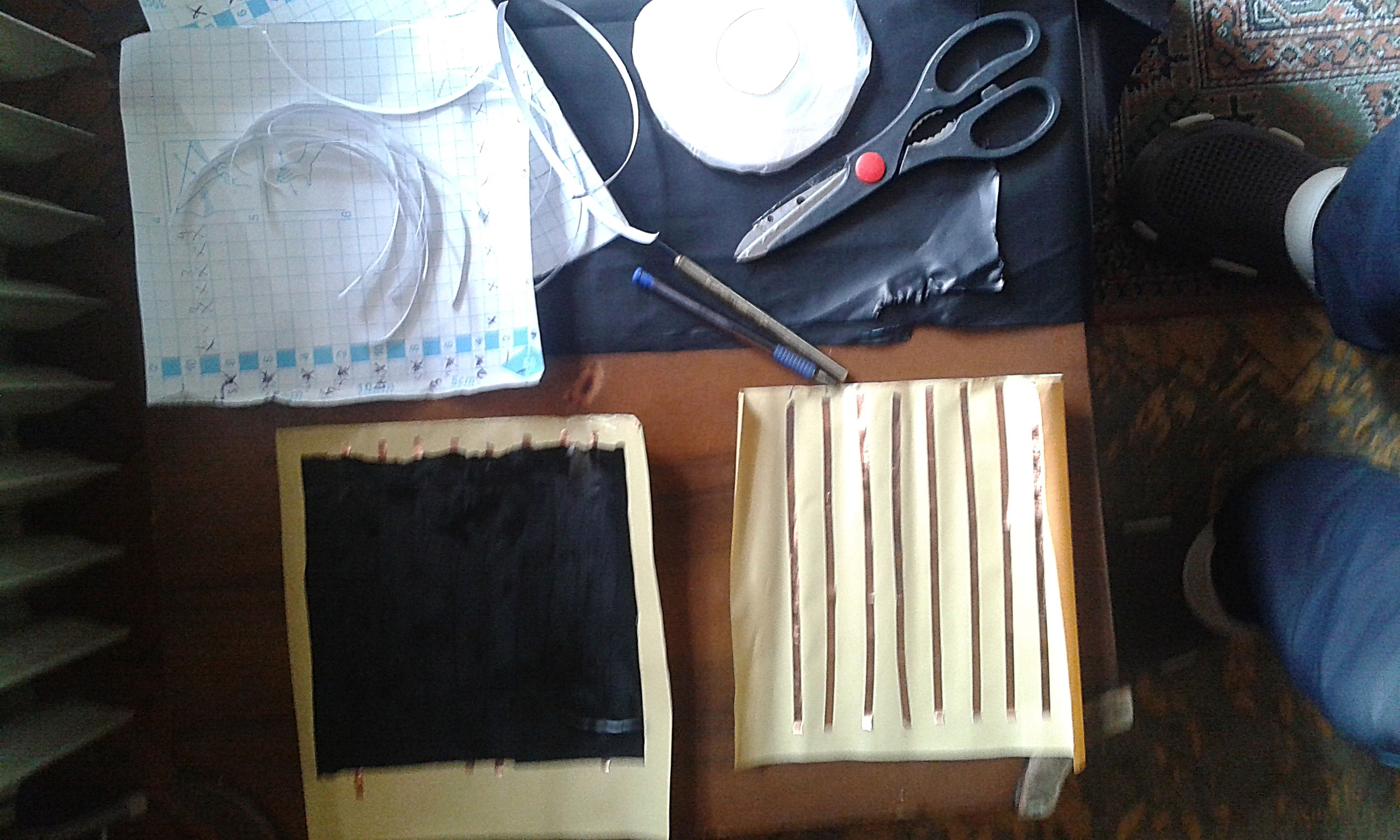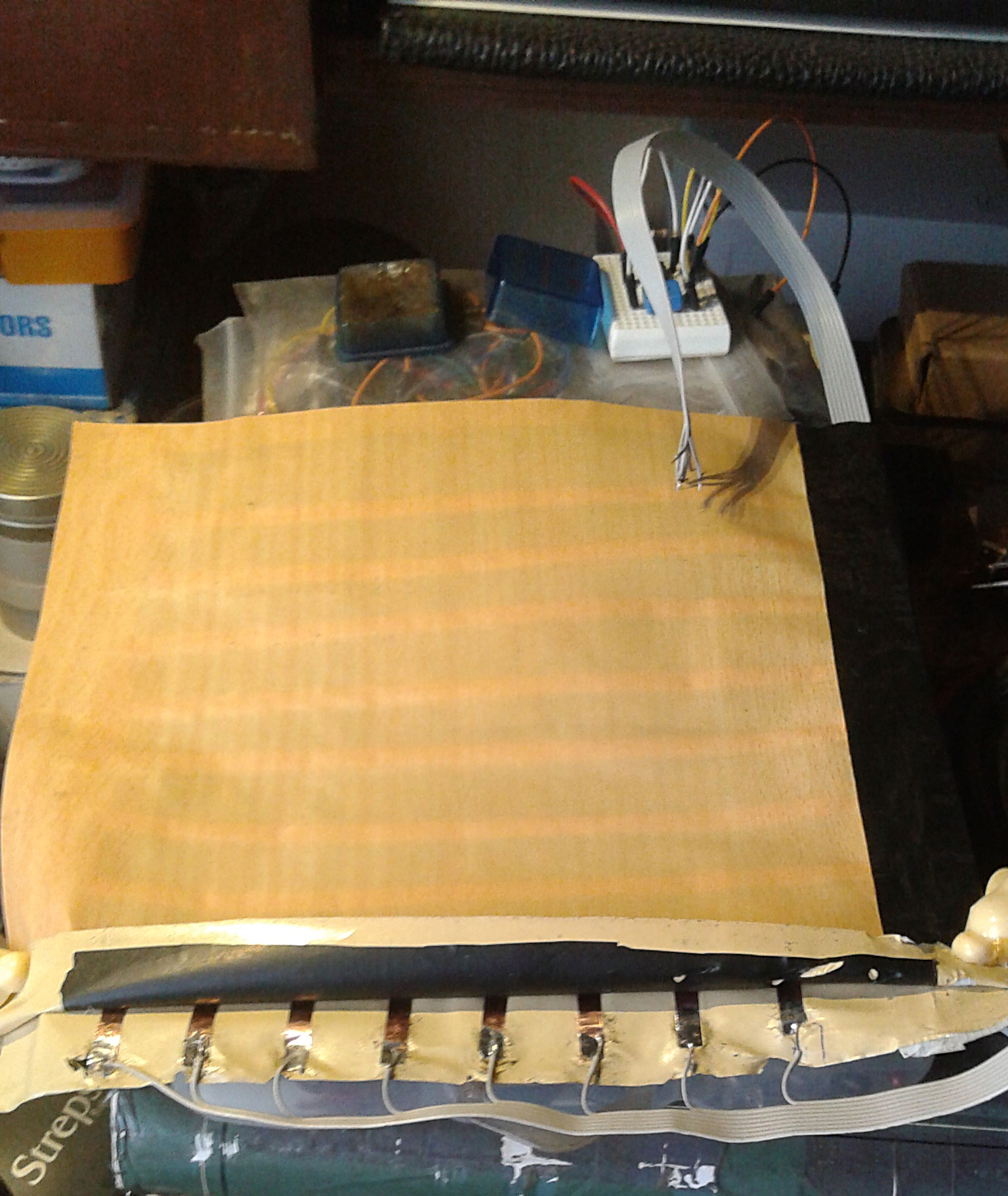As you can see the final skin is very thin and flexible... Can be placed on any part of the robot... Around arms, legs or chest... It can be constructed at any size... The Arduino code for reading the sensor is written by me using a software 12bit ADC for increased sensitivity... I am trying to find a way for visualising the output on Processing.... I will post future improvements.... Arduino code is :

/*
Matrix outputs on digital pins 2-9
Dincer Hepguler 2015
http://borsaci06.com
*/
//include the library

//instantiate an object of this library class; call it "adc"

//Global constants
//const uint8_t pin = A0; //analogRead pin
//const uint8_t pin = A1; //analogRead pin
//const uint8_t pin = A2; //analogRead pin
//const uint8_t pin = A3; //analogRead pin
//const uint8_t pin = A4; //analogRead pin
//const uint8_t pin = A5; //analogRead pin
//constants required to determine the voltage at the pin;
//BE SURE YOU USE THE CORRECT ONE OF THESE WHEN CALCULATING THE VOLTAGE FROM A READING! Take notes of how these constants are used below.

// the setup routine runs once when you press reset:
void setup() {
// initialize serial communication at 9600 bits per second:
Serial.begin(57600);
//pin definitions
pinMode(A0,INPUT);
pinMode(A1,INPUT);
pinMode(A2,INPUT);
pinMode(A3,INPUT);
pinMode(A4,INPUT);
pinMode(A5,INPUT);
pinMode(A6,INPUT);
pinMode(A7,INPUT);
pinMode(2,OUTPUT);
pinMode(3,OUTPUT);
pinMode(4,OUTPUT);
pinMode(5,OUTPUT);
pinMode(6,OUTPUT);
pinMode(7,OUTPUT);
pinMode(8,OUTPUT);
pinMode(9,OUTPUT);
//digitalWrite(2,HIGH);
//digitalWrite(3,HIGH);
//digitalWrite(4,HIGH);
//digitalWrite(5,HIGH);
//digitalWrite(6,HIGH);
//digitalWrite(7,HIGH);
//digitalWrite(8,HIGH);
//digitalWrite(9,HIGH);
}

// the loop routine runs over and over again forever:
void loop() {
//Local variables
unsigned long num_samples=10;
uint8_t bits_of_precision=12; //bits of precision for the ADC (Analog to Digital Converter)
float V; //Voltage calculated on the analog pin

// read the input on analog pins
// print out the values you read as CSV

Serial.print(sensorValue0);
Serial.print(',');
Serial.print(sensorValue1);
Serial.print(',');
Serial.print(sensorValue2);
Serial.print(',');
Serial.print(sensorValue3);
Serial.print(',');
Serial.print(sensorValue4);
Serial.print(',');
Serial.print(sensorValue5);
Serial.print(',');
Serial.print(sensorValue6);
Serial.print(',');
Serial.print(sensorValue7);
Serial.println();
delay(100);        // delay in between reads for stability
}

## Comment viewing options